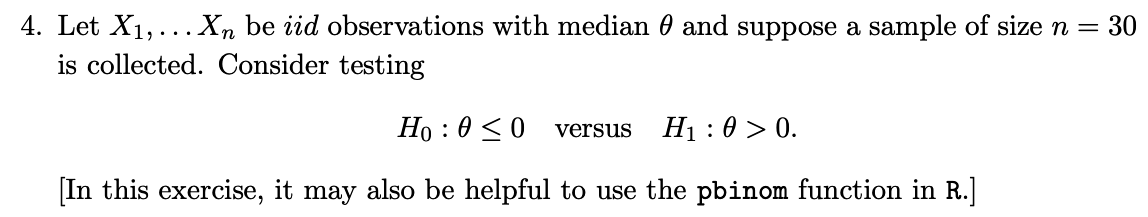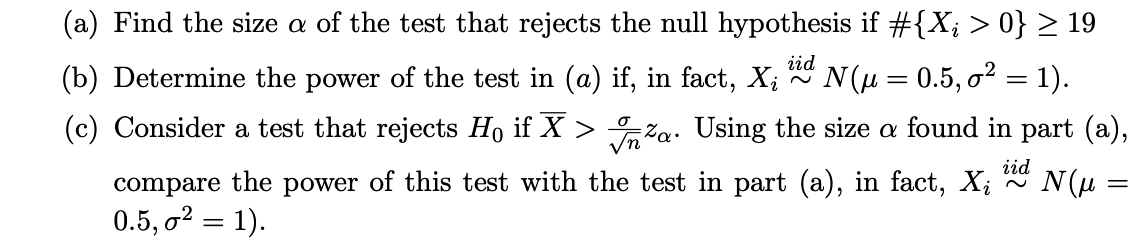# (Solved): 4. Let X1,Xn be iid observations with median and suppose a sample of size n=30 is coll ...4. Let be observations with median and suppose a sample of size is collected. Consider testing (a) Find the size of the test that rejects the null hypothesis if (b) Determine the power of the test in if, in fact, . (c) Consider a test that rejects if . Using the size found in part (a), compare the power of this test with the test in part , in fact,

We have an Answer from Expert